Courses

# OP AMP MCQ Level – 1

## 10 Questions MCQ Test Topic wise Tests for IIT JAM Physics | OP AMP MCQ Level – 1

Description
This mock test of OP AMP MCQ Level – 1 for Physics helps you for every Physics entrance exam. This contains 10 Multiple Choice Questions for Physics OP AMP MCQ Level – 1 (mcq) to study with solutions a complete question bank. The solved questions answers in this OP AMP MCQ Level – 1 quiz give you a good mix of easy questions and tough questions. Physics students definitely take this OP AMP MCQ Level – 1 exercise for a better result in the exam. You can find other OP AMP MCQ Level – 1 extra questions, long questions & short questions for Physics on EduRev as well by searching above.
QUESTION: 1

### In one of the following circuits, negative feedback does not operate for a negative input. Which one is it ? The Op-Amps are running from supplies.

Solution:

Since diode does not conduct in reverse bias hence, the circuit given in option (c) does not operate for a negative feedback.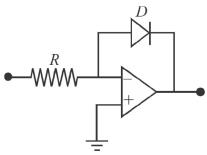QUESTION: 2

### In an integrator, the feedback element is a :

Solution:

Integrator circuit is as shown below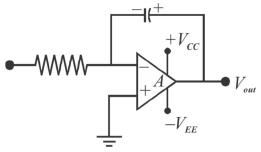QUESTION: 3

### The input and output impedance of the amplifier in given figure, is, (The Op-amp data sheet gives Zin = 2MΩ, ZOut = 75Ω and Aol = 200000).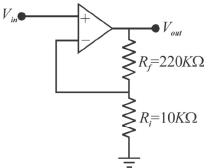Solution:

The attenuation B, of the feedback circuit is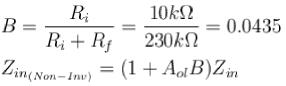= [1 + 200000(0.0435)](2MΩ)
= 17.4GΩ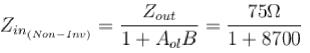= 8.6Ω
The correct answer is: 17.4GΩ; 8.6/mΩ

QUESTION: 4

The frequency at which the open loop gain is equal to 1 is called.

Solution:

It is called the unity-gain frequency
The correct answer is: The unity-gain frequency

QUESTION: 5

In the differentiating circuit given in the figure, the function of R1 is to: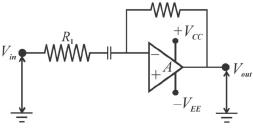Solution:

R1 maintains the high input impedance.
The correct answer is: Maintain high input impedance

QUESTION: 6

Phase shifts through an Op -Amp is caused by.

Solution:

The internal RC circuit provides the phase shift through any amplifier
The correct answer is: the internal RC circuit

QUESTION: 7

For the amplifier shown in figure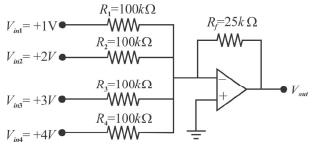Solution:

Since the input resistors are equal;
R= 100/kΩ
The output voltage is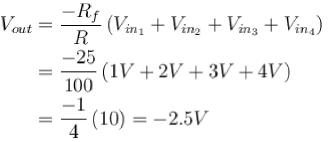Whereas, the input average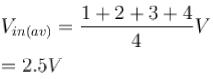Shows that average of the input value is the same magnitude as Vout but of opposite sign.
The correct answer is: The average of the input values is the same magnitude as Vout but of opposite sign

QUESTION: 8

The midrange open-loop gain of an Op-Amp :

Solution:

It extends from 0Hz to the upper critical frequency.
The correct answer is: extends from 0Hz to the upper critical frequency

QUESTION: 9

Figure shows a practical integrator with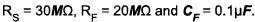If a step (dc) voltage of +3V is applied as input for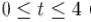( f in second), the output voltage is.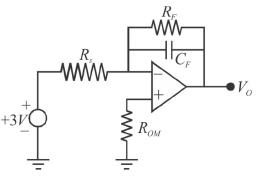Solution: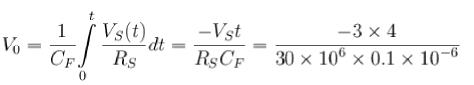= -4V
The correct answer is: a ramp function of -4V

QUESTION: 10

The circuit shown is based on ideal operational amplifiers. It acts as a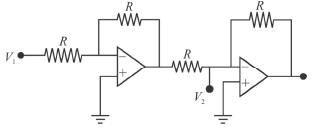Solution:

A buffer amplifier (sometimes simply called a buffer) is one that provides electrical impedance transformation from one circuit to another, with the aim of the signal source being unaffected by (“buffered from”) whatever currents that the load may produce.
The correct answer is: buffer amplifier